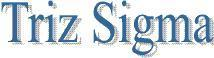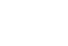Control Charts online advertising Introduction A Control Chart is a tool you can use to monitor a process. It graphically depicts the average value and the upper and lower control limits (the highest and lowest values) of a process. All processes have some form of variation. A Control Chart helps you distinguish between normal and unusual variation in a process. If you want to reduce the amount of variation in a process, you need to compare the results of the process with a standard. Variation can exist for two reasons: Common causes are flaws inherent in the design of the process. Special causes are variations from standards caused by employees or by unusual circumstances or events. Control charts for measurements X-bar charts R charts Control charts for compliance items P charts c chartsX Bar Chart Monitor process location (center) Decide on the quality to be measured. Determine a sample size. Gather 20 to 30 samples. Compute the sample average for each sample. Compute the sample range for each sample. Determine the average sample mean for all samples. Determine the average sample range (or sample standard deviation) for all samples. Determine the value of A2 ( for range) or A3. (for standard deviation). Compute the UCL and the LCLMonitor process variation Decide on the quality to be measured. Determine a sample size. Gather 20 to 30 samples. Compute the sample range for each sample. Determine the average sample mean for all samples. Using the size of the samples, determine the values of D3 and D4. Compute the UCL and the LCL